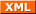# Christoph's last Weblog entries

### Entries tagged "csawctf".

3rd October 2017

A short while ago, FAUST participated in this year's CSAW qualification and -- as usual -- I was working on the Crypto challenges again. The first puzzle I worked on was called "Another Xor" -- and, while there are quite some write ups already our solution was somewhat different (maybe even the intended solution given how nice things worked out) and certainly interesting.

The challenge provides a cipher-text. It's essentially a stream cipher with key repeated to generate the key stream. The plain-text was plain + key + checksum.

p = this is a plaintextThis is the keyfa5d46a2a2dcdeb83e0241ee2c0437f7
k = This is the keyThis is the keyThis is the keyThis is the keyThis i


## Key length

Our first step was figuring out the key length. Let's assume for now the key was This is the key. Notice that the key is also part of the plain-text and we know something about its location -- it ends at 32 characters from the back. If we only take a look at the encrypted key it should have the following structure:

p' = This is the key
k' = he keyThis is t


The thing to notice here is that every character in the Key appears both in the plain-text and key stream sequence. And the cipher-text is the XOR (⊕) of both. Therefore XOR over the cipher-text sequence encrypting the key should equal 0 (⊕(p') ⊕ ⊕(k') = 0). So remove the last 32 characters and find all suffixes that result in a XOR of 0. Fortunately there is exactly one such suffix (there could be multiple) and therefore we know the key size: 67.

To put it in code, this basically is the function we implemented for this:

def calculate(ciphertextcandidate):
accumulator = 0
for char in ciphertextcandidate:
accumulator = accumulator ^ char


Which, for the matching plain-text and key-stream fragments is equal (due to the XOR encryption) to

def calculate(plainfragment, keyfragment):
accumulator = 0
for i in range(len(plainfragment):
accumulator = accumulator ^ (plainfragment[i] ^ keyfragment[i])


Now XOR lets us nicely reorder this to

def calculate(plainfragment, keyfragment):
accumulator = 0
for i in range(len(plainfragment):
accumulator = accumulator ^ (plainfragment[i] ^
keyfragment[(i + 6) % len(plainfragment)])


And, as plainfragment[i] and keyfragment[(i + 6) % len(plainfragment)] are equal for the plain-text range encoding the key this becomes

def calculate(plainfragment, keyfragment):
accumulator = 0
for i in range(len(plainfragment):
accumulator = accumulator ^ 0


Or simply 0 if the guess of the cipher-text range is correct.

## Key recovery

Now the nice thing to notice is that the length of the key (67) is a prime (and 38, the plain-text length, is a generator). As a result, we only need to guess one byte of the key:

Assume you know one byte of the key (and the position). Now you can use that one byte of the key to decrypt the next byte of the key (using the area where the key is part of the plain-text). Due to the primeness of the key length this allows recovery of the full key.

Finally you can either print all 256 options and look for the one that looks reasonable or you can verify the md5sum which will give you the one valid solution, flag{sti11_us3_da_x0r_for_my_s3cratz}.

## Code


cipher = b"'L\x10\x12\x1a\x01\x00I[P-U\x1cU\x7f\x0b\x083X]\x1b'\x03\x0bR(\x04\r7SI\n\x1c\x02T\x15\x05\x15%EQ\x18\x00\x19\x11SJ\x00RV\n\x14YO\x0b\x1eI\n\x01\x0cE\x14A\x1e\x07\x00\x14aZ\x18\x1b\x02R\x1bX\x03\x05\x17\x00\x02\x07K\n\x1aLAM\x1f\x1d\x17\x1d\x00\x15\x1b\x1d\x0fH\x0eI\x1e\x02I\x01\x0c\x15\x00P\x11\\PXPCB\x03B\x13TBL\x11PC\x0b^\tM\x14IW\x08\rDD%FC"

def keycover(guess):
key = dict()
pos = 38
key = guess

for i in range(67):
newpos = (pos % 67) + 38
key[newpos] = xor(cipher[pos:], key[pos])
pos = newpos

try:
return b''.join([ key[i] for i in range(38, 105, 1) ])
except:
return b'test'

for guess in range(256):
keycand = keycover(bytes([guess]))

plaincand = xor(cipher, repeat(keycand, len(cipher)))

if md5(plaincand[:-32]).hexdigest().encode() == plaincand[-32:]:
print(keycand, plaincand)

Tags: crypto, csawctf, ctf, faust, security, writeup.Created by Chronicle v4.6